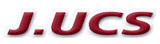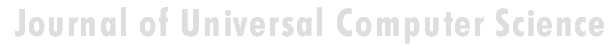Search
 Submission Procedure share: |available in:   PDF (185 kB) PS (172 kB)

get:
 Similar Docs BibTeX Write a comment
get:

DOI:   10.3217/jucs-014-06-0845

### Computable Riesz Representation for Locally Compact Hausdorff Spaces

Hong Lu (Nanjing University, China)

Klaus Weihrauch (University of Hagen, Germany)

Abstract: By the Riesz Representation Theorem for locally compact Hausdorff spaces, for every positive linear functional I on K(X) there is a measure μ such that I(f) =∫ f dμ where K(X) is the set of continuous real functions with compact support on the locally compact Hausdorff space X. In this article we prove a uniformly computable version of this theorem for computably locally compact computable Hausdorff spaces X. We introduce a representation of the positive linear functionals I on K(X) and a representation of the Borel measures on X and prove that for every such functional I a measure μ can be computed and vice versa such that I(f) = ∫ f dμ.

Keywords: Hausdorff spaces, Riesz representation theorem, computable analysis, computable topology

Categories: F.0, F.1.0, F.1.1